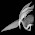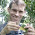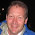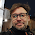## Wednesday, November 28, 2012

### Fitting the λ model in phylogenetic regressions using various functions

I am trying to implement Liam Revell's suggestion on the evaluation of Pagel's lambda simultaneous to fitting PGLS to minimize the effects of wrong model selection (OLS vs. PGLS) on species data (i.e. Revell 2010, Methods in Ecology and Evolution 1: 319-329).Does anyone know if this kind of simultaneous optimization of lambda and PGLS parameters is presently implemented in R? The obvious place would be phytools, but I could not find anything similar in that package.

In fact, this is possible using multiple functions in several packages. Unfortunately, it is not always completely obvious - so the question is (in my opinion) fully justified! Specifically, this can be done using nlme::gls, caper::pgls, and (indeed) phytools::phyl.resid. Here's my summary (modified from my response) of how (which I repeat here in the hope that it will help folks in future when they are searching for information on this topic):

# using ape & nlme
library(ape); library(nlme)
# assuming your data are contained in named vectors x & y
y<-y[names(x)]
fit<-gls(y~x,data.frame(x,y),correlation=corPagel(1,tree, fixed=FALSE),method="ML")
# assuming your data are in data frame X, with column names
# var1, var2, etc.; for example:
fit<-gls(var1~var2+var3,X,correlation=corPagel(1,tree, fixed=FALSE),method="ML")

# using caper
library(caper)
# assuming your data are in named vectors x & y
y<-y[names(x)]
X<-data.frame(x,y,Species=names(x))
fit<-pgls(y~x,comparative.data(tree,X,"Species"), lambda="ML")

# using phytools
library(phytools)
# assuming your data are in named vectors x & y
fit<-phyl.resid(tree,x,y,method="lambda")

I would generally recommend using gls & pgls over phyl.resid, because they "play well" with generic functions such as summary and residuals, with the following caveats (1) phyl.resid may be a little easier to use (because it takes standard arguments, no functions or formulae); and (2) phyl.resid (for some reason) seems to run a little faster, especially for large trees. (For instance, it took only 45s on a tree with 1000 tips while gls took over a minute and pgls over 4 minutes. This could be important for investigators repeating the same analysis on many trees - for instance, a sample from the posterior distribution of a Bayesian phylogeny inference run.)

1.Hi Liam,

I want to get an overall p-value for PGLS regression with coestimation of lambda. It's clear how to do this with anova() and nlme's gls(), i.e. test against a X ~ 1 null model.

However, if I wanted to use phyl.resid() how would I do that? I don't have a large tree, but I do have thousands of regressions to run.

Thanks!
Dan Fulop.

1.Hi Dan.

I would actually recommend you just use gls in the nlme package.

This would look something like the following (for data vectors x & y, and phylogeny, tree):

fit<-gls(y~x,data=data.frame(x,y),correlation= corPagel(1,tree),method="ML")
anova(fit)
resid<-residuals(fit)

This should give the same fit, likelihood, and residuals as phyl.resid, and you can also get a P-value for the model using anova.

If your tree is not ultrametric there is an extra step:

vf<-diag(vcv(tree))
fit<-gls(y~x,data=data.frame(x,y),correlation=corPagel(1,tree),method="ML",weights=varFixed(~vf))
anova(fit)
resid<-residuals(fit)

Good luck. Liam

2.This comment has been removed by the author.

3.This comment has been removed by the author.

4.Dear Liam,

Is there a way to weigh pgls {caper} models by samle size?

Thanks,
Jasmine

1.I'd like to second this + would be extremely useful!

5.Dear Liam,

Im writing you because id like to know more exactly what its meant when you wrote:

In GLS..."we have down-weighted each observation for Y (and corresponding row of X) depending on the correlation of its residual error with the other observations in our set."

So far, I think this consists in comparing the residuals of the correlation obtained between two variables with the residuals of the correlation obtained between the same variables simulated along the tree like they followed a brownian motion. If this is right, I could not see how the similarity of the obtained residuals with the expected residuals actually leads to a "downweight" of each observation. Is there any place where these calculations can be followed in R?

Im sorry I could no grasp that from your paper your 2010 paper "phylogenetic signal and linear regressions on species data." Despite it is probably actually there.

Agus

6.Dear Liam,

I am attempting to perform a multiple phylogenetic regression using nlme and ape with the following command style

fit<-gls(var1~var2+var3,X,correlation=corPagel(1,tree, fixed=FALSE),method="ML")

I have 3 continuous and 3 categorical variables in my specific dataset. When I try to run this analysis with my data using the above approach, I get the following error

Error in corFactor.corStruct(object) :
NA/NaN/Inf in foreign function call (arg 1)

Funnily enough if I run the model with fewer X variables the model works, but not always and it seems to depend on which combination of variables I include. On these different occasions I either get the same error message (as above) or I get a new message, (eg. "Error in eigen(val) : infinite or missing values in 'x'" or Error in gls(freq_urban_trans ~ freq_surround_trans + fnest + fhabitat + :
false convergence (8)). Additionally, if I set the starting lambda value to 0 the model runs, but produces a negative estimate of lambda.

I have spent some time trying to resolve the issue via searching help lists, but have not been able to resolve the issue. I was wondering if you have any suggestions for why the more complex model will not run, but a simpler model will.

Finally, my reasons for using this approach over pgls in caper, is that this approach allows me to summarise the data using the anova command and specify the use of Type III (marginal) sum of squares. This is an important issue for me as my categorical variables have > 2 unordered levels and thus using the summary command does not provide information on the overall effect of the variable.

Any suggestions you have for resolving these issues would be greatly appreciated.

melissah

1.Hi Melissah.

I'm not sure, as I don't maintain gls (in nlme) or corPagel (in ape); consequently, I'm not familiar with the error message your referring to. You should try posting this question to the R-SIG-phylo email list.

All the best, Liam

7.Dear Liam!

I would like to obtain confidence ntervals for the parameters from pgls model. I do not mean confidence intervals for lambda, but for intercept and slope, but phylogenetically corrected. I tried to use basic R functions like confint, but it did not work. Maybe you know how to do it?

Best,

Andrzej

8.Dear Liam,

Many thanks for the package and blog, certainly a great contribution to the field.

I would like to test a linear model between a predictor variable (for instance altitude) and a dependent variable (number of flowers). For this, I do have several observations of individuals of 42 species along the predictor variable, but the number of observations do vary per each species and class of the independent variable. In the end, I do have 364 observations unbalanced per each of the 42 species and environment.

My intention is doing a model having the number of flower as dependent variable, the temperature as fixed effect, using species as a random factor and add a phylogenetic correction.

I could do part of this this following your example with GLS, but the fact of having multiple observations per species do not allow running the model and returns the following message:
“number of observations and number of tips in the tree are not equal.”

I would much appreciate if you could guide me on how to overcome this issue and run such model.

Many thanks,
Nicolay

1.Hi Nicolay,

I met the same problem that multple observations per species are in my data. Did you fix the problems? If so, could you please share the solution?

best
Liu

2.Hi Liu,

In the list R-sig-phylo, I was encouraged to try Bayesian mixed modeling using the r packages brms and MCMCglmm. But I am still learning on how to use it and could not solve my problem...

Hope you get better luck.

Cheers,
Nicolay

9.This comment has been removed by the author.

Note: due to the very large amount of spam, all comments are now automatically submitted for moderation.